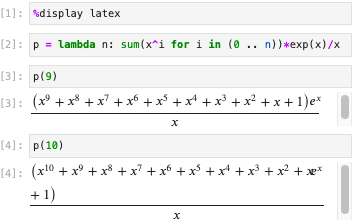# Line break in latex output makes it read wrong

With %display latex, long expressions can read wrong due to weird line breaks.

For example, run for various values of n the following:

p = lambda n: sum(x^i for i in (0 .. n))*exp(x)/x
p(9)  # or more depending on window widthWhy isn't the factor $e^x$ after the parenthesis on the second line instead?

Is this even a SageMath, a Jupyter, or a MathJax issue? Where to report it?

Versions: JupyterLab 2.2.9, SageMath 9.3beta4.

edit retag close merge delete

The automatic "LaTeXification" (more precisely "mathjaxification") works reasonably well ... in "reasonable" cases, but has its limits. which you seem to have reached.

You could manipulate your result to "break" it in manageable parts ; say the denominator and the numerator, the latter being a polynomial which can be broken up in reasonably-sized parts. latex() each of these parts and build a LatexExpr representing correctly your result, using, for example, Latex arraysor align* as needed. In your case, something like LatexExpr(\frac{\array[c]{<lines of the numerator)}{<denominator>}). (Typed from memory, exact syntax to be checked).

Not having your original result prevents me to be more precise or proposing a solution...

My problem is not really to obtain a perfect "LaTeXification", If I want to copy it in a document the width will probably be different anyway, but this is just mistake inducing from me (I just lost 30 minutes this morning trying to understand this particular example, until at one point I resized the window and it went away. I was very sad...)

Sorry for not providing a minimal example. Here is one: (sum(x^i for i in range(34)))*exp(x)/x. If I increase the max degree above 35, a line break occurs (It will depend on your screen real estate).

Once again, aligning the $e^x$ with the last line instead of the first would solve the ambiguity.

Why not use something like:

f = (sum(x^j for j in range(34)))*exp(x)/x
print(latex(f))


which gives:

\frac{{\left(x^{33} + x^{32} + x^{31} + x^{30} + x^{29} + x^{28} + x^{27} + x^{26} + x^{25} + x^{24} + x^{23} + x^{22} + x^{21} + x^{20} + x^{19} + x^{18} + x^{17} + x^{16} + x^{15} + x^{14} + x^{13} + x^{12} + x^{11} + x^{10} + x^{9} + x^{8} + x^{7} + x^{6} + x^{5} + x^{4} + x^{3} + x^{2} + x + 1\right)} e^{x}}{x}


and there is no line break. When using %display you insist to display the stuff...

Because I want to display the stuff. I think a nice output makes it a lot simpler to understand a result. The latex output is usually superior to a simple print in this regard. Except when it's actively trying to confuse me, like in the first example I provided.

1

I allowed myself to edit the question to use the simpler example.

Sort by » oldest newest most voted

Reported upstream (to MathJax) as

A workaround might be to disable line wrapping.

This is what SageCell and CoCalc do, apparently.

Not sure how one can disable MathJax line wrapping:

• in a given Jupyter sheet
• in a given Jupyter installation
• for a given Jupyter kernel
• ...
more